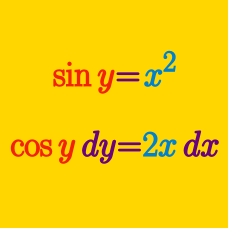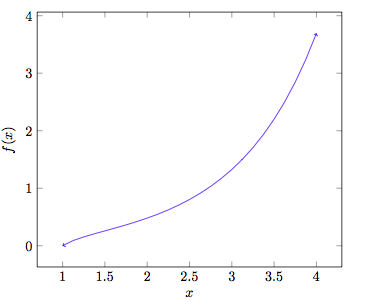Calculus

# Implicit Differentiation - Exponential and Logarithmic Functions

If $$\displaystyle y^{10}+x^{5}y^{6}=2+ye^{x^2},$$ what is $$\displaystyle \frac{dy}{dx}?$$

If $$\displaystyle e^{\frac{x}{y}}=4x-6y,$$ what is $$\displaystyle \frac{dy}{dx}?$$

What is the equation of the tangent line to $$\ln (x+4y)+3e^y=3$$ at the point $$(1,0)?$$If $$y=(\ln x)^x$$, what is the derivative of $$y$$ at $$x=e$$?

Given $$e^{2xy} = y^2$$, what is the value of $$\frac{dy}{dx}$$ at $$(x,y) = (0,1)$$.

×